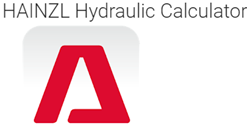# 7- Best hydraulic calculator apps for android and their features

1. Hydraulic system calculator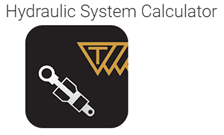This app will be very useful for people who work with hydraulic cylinders, pumps, motors, and pipes. By using this app we can design single-acting and double-acting cylinders. The area and volume of the cylinder can be calculated by entering the dimensions of the cylinder and parameters like pressure, oil flow. We can also calculate velocity, extraction, and retraction forces, time, and outflow by this app. By using this app we can calculate the flow rate, pressure, displacement, speed, torque, and power in the motor section. We can also calculate the displacement, flow rate, electric motor power, and total efficiency in the pump section. It can also calculate the pressure drop around an orifice and also it can calculate the piping parameters like velocity, cross-sectional area and Reynolds number can be calculated by using absolute or kinematic viscosity.

2. Hydraulic calculator freeThis app can be used for the calculation of pressure drop in the design of liquid, gas, two-phase fluid pipelines, taking account of inline fittings will be carried out. The friction factor is calculated by using Poiseuille’s equation for laminar flow and Cole-brooks equation for transitional and turbulent flows. It can also be used for unit conversion of mass flow rate, volume flow rate, temperature, pressure, density.

3. Hydraulic slide calculator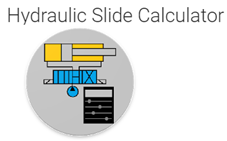This app can be used to do rapid hydraulic calculations to size hydraulic components during hydraulic system design. This app is not like other hydraulic calculator apps and the reason for that is it won’t require additional inputs to get answers, it would update itself as sliders are swiped and when the buttons are tapped. By using this app we can calculate the size of hydraulic cylinders, hydraulic pumps, and hydraulic motors.

4. Hydraulic calc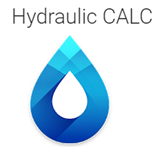This app will be very useful for engineers because it can do many hydraulic calculations. It also has many formulas, theories, and coefficients related to hydraulics. It can be considered as the best flow calculator.

5. Hydraulic calculationBy using this app we can do the hydraulic calculation of the pipeline section in the installation of the energy metering unit. It can do the calculations like volumetric flow rate of water, the kinematic viscosity of water, Reynolds number, pressure drop on the straight section, loss of pressure in the diffuser, total head-loss, the drag coefficient of the expansion, and lot more.

6. Hydraulic calculations plus hydraulic cylinder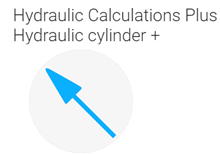This app will be very helpful to calculate hydraulic cylinder, pumps, motors, fluid pipes, leakage, and more. This app can be used to do the following calculations like force, pressure, area, hydraulic cylinder calculations, the pressure drop through a straight pipe, the pressure drop through an orifice, temperature change due to pressure drop, leakage flow in a radial gap. It can also do hydraulic pump calculations like calculation of output flow rate, displacement pump speed, shaft power, etc. it can also do hydraulic motor calculations like oil flow, displacement, input and output power, motor torque, and lot more.